# LeetCode-位運算相關題解

### LeetCode-191 二進制位1的個數

LeetCode上第 191 號問題：編寫一個函數，輸入是一個無符號整數，返回其二進制表達式中數字位數為 ‘1’ 的個數。

class Solution:
def hammingWeight(self, n):
res = 0
while n != 0:
res += 1
n &= (n - 1)
return res

def hammingWeight2(self, n):
res = 0
while n != 0:
res += (n & 1)
n = n >> 1
return res


### LeetCode-231 2的冪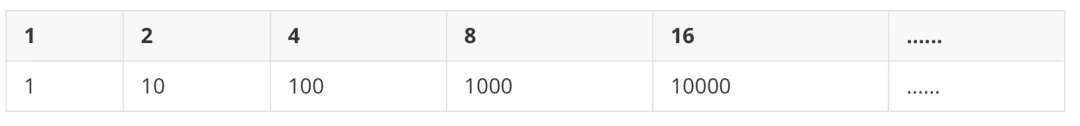def isPowerOfTwo(n):
res = 0
while n != 0:
res += (n & 1)
n >>= 1
return res == 1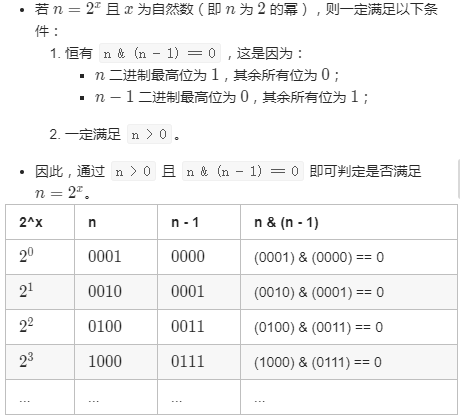class Solution:
def isPowerOfTwo(self, n: int) -> bool:
return (n > 0) and ((n & (n - 1)) == 0)


### LeetCode-201. 閉區間范圍內數字按位與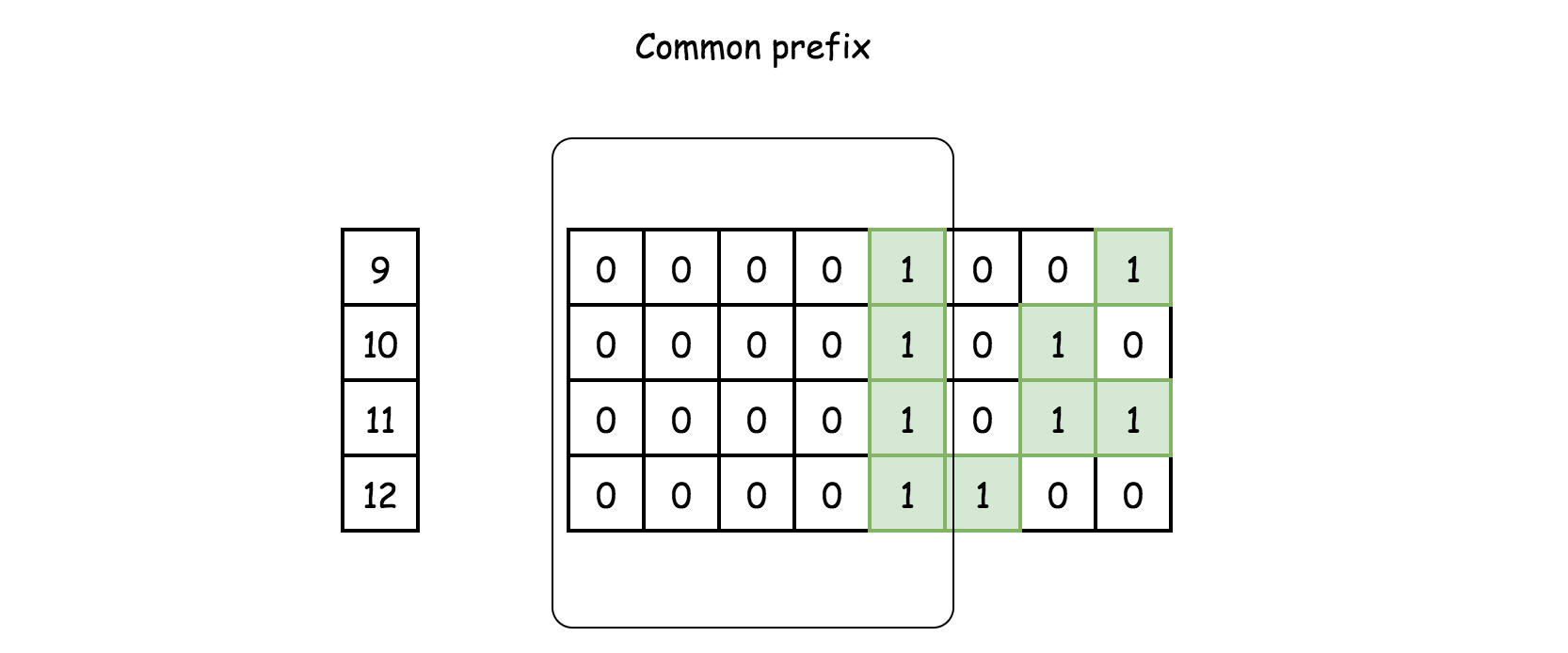1. 使用191的方法 Brian Kernighan 算法 n & (n-1)
class Solution:
def rangeBitwiseAnd(self, m: int, n: int) -> int:
while m < n:
# turn off rightmost 1-bit
n = n & (n - 1)
return m & n

1. 找m, n 的最高位1出現的位置 ， 如果不相等，則返回0，如果相等，則找公共前綴。B站視頻-位運算練習【LeetCode】

### LeetCode-187.重復的DNA序列

輸入：s = "AAAAACCCCCAAAAACCCCCCAAAAAGGGTTT"


# 普通解法
class Solution:
def findRepeatedDnaSequences(self, s: str) -> List[str]:
d = {}
for i in range(len(s) - 9):
k = s[i: i+10]
if k in d:
d[k] = True
else:
d[k] = False

return [*filter(lambda x: d[x], d)]


### LeetCode-36.只出現一次的數字

輸入: [2,2,1]



a b a⊕b
1 0 1
1 1 0
0 0 0
0 1 1
class Solution:
def singleNumber(self, nums: List[int]) -> int:
# 非空數組暫時不用判斷
from functools import reduce
return reduce(lambda a, b: a ^ b, nums)


### LeetCode-137.只出現一次的數字 II

輸入: [2,2,3,2]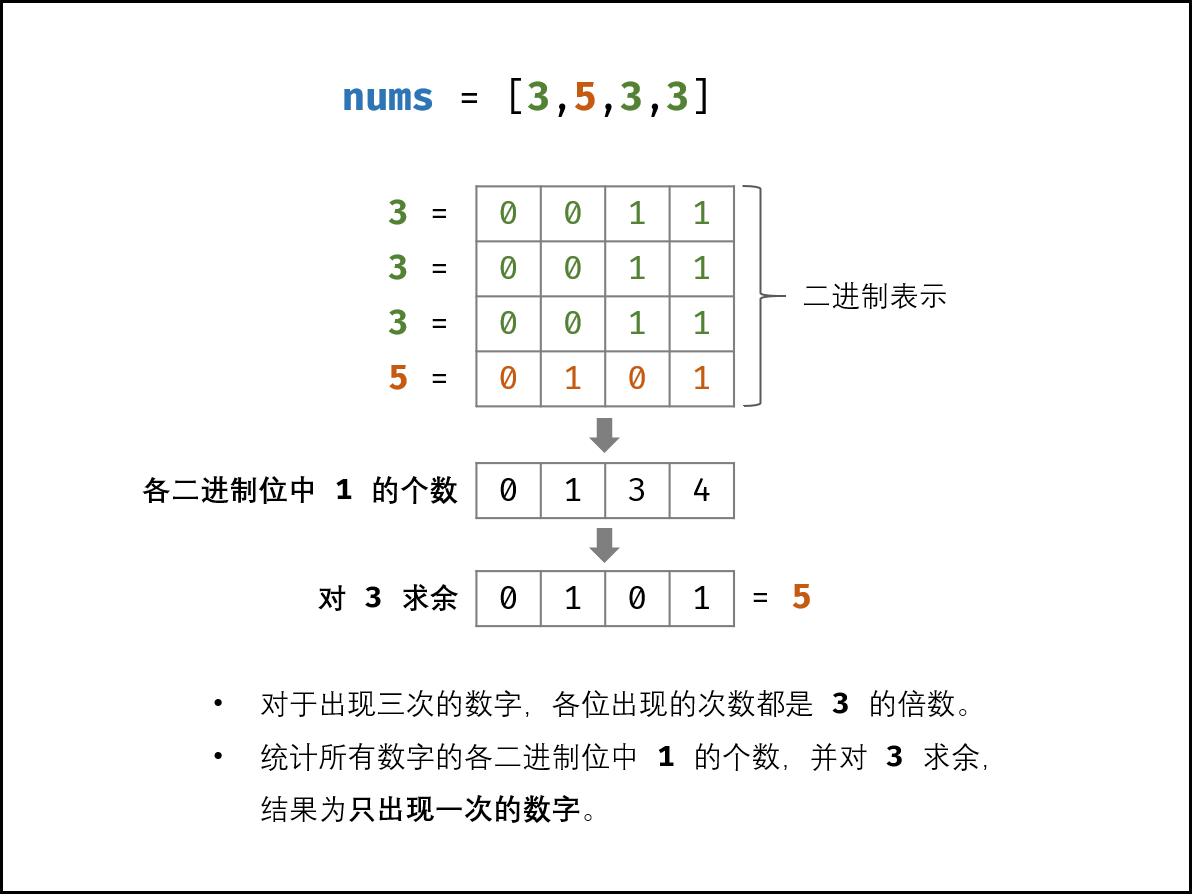3×(a+b+c)?(a+a+a+b+b+b+c)=2c 也可以應用在上一題

## 普通解法
class Solution:
def singleNumber(self, nums):
return (3 * sum(set(nums)) - sum(nums)) // 2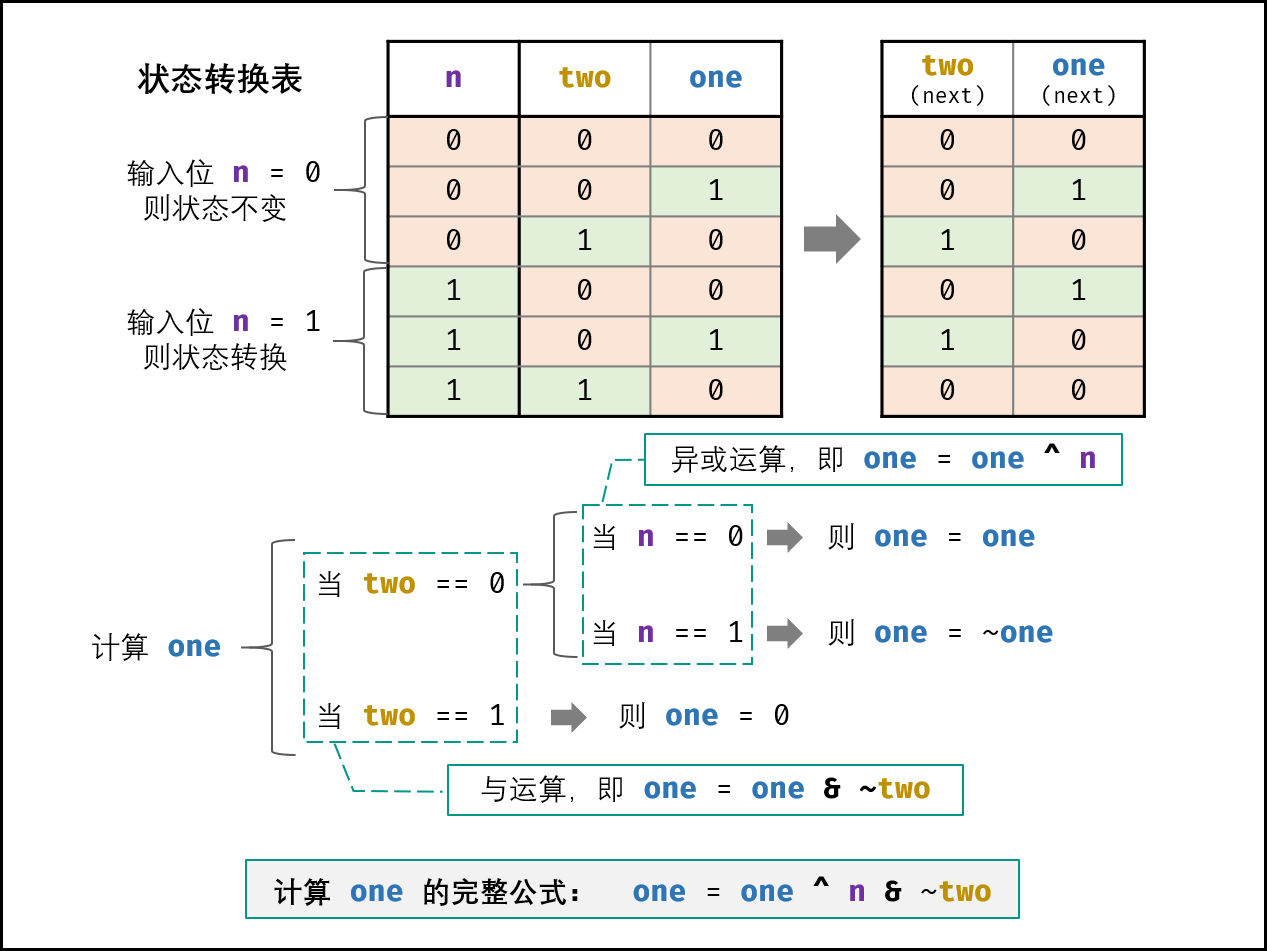class Solution:
def singleNumber(self, nums: List[int]) -> int:
ones, twos = 0, 0
for num in nums:
ones = ones ^ num & ~twos
twos = twos ^ num & ~ones
return ones


### LeetCode-260. 只出現一次的數字 III

輸入: [1,2,1,3,2,5]



1. 結果輸出的順序并不重要，對于上面的例子， [5, 3] 也是正確答案。
2. 你的算法應該具有線性時間復雜度。你能否僅使用常數空間復雜度來實現？

class Solution:
def FindNumsAppearOnce(self, nums):
length = len(nums)
if length <= 0:
return nums
result = 0
# 先將所有數子異或得到一個值
for num in nums:
result ^= num
# 找到這個值最低位二進制位1的位置，根據這個位置來區分兩個數組，分別異或求出只出現一次的數字
firstBitIndex = self.FindFirstBit(result)
n1, n2 = 0, 0
for num in nums:
if self.IsSameBit(num, firstBitIndex):
n1 ^= num
else:
n2 ^= num
return n1, n2

def FindFirstBit(self, num):
indexBit = 0
while num & 1 == 0:
indexBit += 1
num = num >> 1
return indexBit

def IsSameBit(self, num, indexBit):
num = num >> indexBit
return num & 1

# 解放2
class Solution2:
def FindNumsAppearOnce(self, nums):
length = len(nums)
if length <= 0:
return []

diff = 0
for i in nums:
diff ^= i

n1, n2 = 0, 0
minDiff = self.getMinDiff(diff)
for num in nums:
if minDiff & num == 0:
n1 ^= num
n2 = diff ^ n1
return n1, n2

def getMinDiff(self, num):
# 保留一個低位是1的數字
# 取負號其實就是先取反，再加 1，需要 補碼 的知識。最后再和原數相與就會保留最低位的 1。比如 1010，先取反是 0101，再加 1，就是 0110，再和 1010 相與，就是 0010 了
return num & (-num)


### 如何得到二進制位只有一個1的數，幾種方法

diff &= -diff ; 這里 的做法。

diff = (diff & (diff - 1)) ^ diff; 這里 的做法

n & (n - 1) 的操作在 191 題 用過，它可以將最低位的 1 置為 0。比如 1110，先將最低位的 1 置為 0 就變成 1100，然后再和原數 1110 異或，就得到了 0010

diff = xor & ~(diff - 1) 這里 的做法

mask=1


### 推到1到N連續自然數的異或值

1 1=1; 1
2 1^2=3; n+1
3 1^2^3=0; 0
4 1^2^3^4=4; n
5 1^2^3^4^5=1; 1
6 1^2^3^4^5^6=7; n+1
7 1^2^3^4^5^6^7=0; 0
8 1^2^3^4^5^6^7^8=8; n
... ... ...

def main(n):
k = n % 4
res = {
0: n,
1: 1,
2: n + 1,
3: 0
}

return res[k]


# 根據異或的性質推到可以得到如下結果，則有化簡為1->n之間的異或
f([m, n]) = f([1, n])^f([1, m-1])



### 參考資料

posted @ 2020-07-17 23:51  JonPan  閱讀(59)  評論(0編輯  收藏